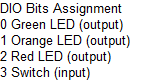The following information is for the EMANT300, EMANT380

# Exercise 8 – Decision Making Statements

## Objective

• Learn If statement

• Revise analog input

• Learn digital output

Night lights are normally used in children's bedrooms to reduce their fear of the dark and to provide some light in a darkened room. But even without children, a home should use night lights for safety. The lights use built-in photo sensors so that they turn on only at night.

In this exercise, we will program the light sensor and the Green LED to create a simple night light. We use the digital outputs to turn on and off the LED. To do this we will use the if statement

1.Open the Visual Basic solution VB_2010_Solution.sln.

2. Set the Ifled project as the startup project.

3. View the project code by opening the project's Module1.vb

4.If you are using the EMANT380 Bluetooth DAQ, change the parameter to False and COM5 to the COM port you are using, See exercise 1 step 4.

``DAQ.Open(False,"COM5")``

5. Press Ctrl+F5 to Start without Debugging to run the program. Enter the Lux threshold. Choose a Lux threshold that is between the light intensity when the Photodiode is covered and when it is not. Observe the Green LED turning on when the light level drops below the threshold. The Light Intensity measured in Lux is also displayed.

6. Press any key to return to the development environment.

## Program 8.1 Night Light

``````Imports Emant
Module Module1
Sub Main()
Dim volt, lux, compare As Double
Dim input As String
Console.WriteLine("Light Threshold in Lux")
input = Console.ReadLine
compare = Convert.ToDouble(input)
Dim DAQ As Emant300 = New Emant300
DAQ.Open(True,"COM5")
For i = 0 To 9
volt = DAQ.ReadAnalog(Emant300.AIN.AIN0, Emant300.AIN.COM)
lux = 1333 * volt
Console.WriteLine(lux)
If lux < compare Then
DAQ.WriteDigitalBit(0,True)
Else
DAQ.WriteDigitalBit(0,False)
End If
DAQ.Delay(1000)
Next
DAQ.Close()
End Sub
End Module``````If you are writing this program from scratch, in order to use the Emant300 class, the class library Emant300.dll must be added to the references folder. See exercise 5.

## Relational and Boolean Operators

Relational Operators are used to compare data. They are used to determine the result of a comparison to True or False

``lux < compare``

The following table summarizes some of Visual Basic’s relational and boolean operators, listing the operator categories in order of precedence from highest to lowest. Operators in the same category have equal precedence.

 Category Expression Description Relational and type testing x < y Less than x > y Greater than x <= y Less than or equal x >= y Greater than or equal x Is T Return true if x is a T, false otherwise Equality x == y Equal x != y Not equal Logical AND x And y Integer bitwise AND, boolean logical AND Logical XOR x Xor y Integer bitwise XOR, boolean logical XOR Logical OR x Or y Integer bitwise OR, boolean logical OR

## If statement

The If statement selects a statement for execution based on the value of a boolean expression.

If boolean-expression Then statement End If
If boolean-expression Then statement Else statement End If

An if statement is executed as follows:

1. The boolean-expression is evaluated.

2. If the boolean expression yields true, control is transferred to the statement following Then. When and if control reaches Else, control is transferred to End If, the end point of the if statement.

3. If the boolean expression yields false and an Else part is present, control is transferred to the statement following Else.

4. If the boolean expression yields false and if an Else part is not present, control is transferred to End If, the end point of the if statement.

``````If lux < compare Then
DAQ.WriteDigitalBit(0,True)
Else
DAQ.WriteDigitalBit(0,False)
End If
``````In our example, assuming compare = 100 and two measurements are taken, one with lux = 50 and the second with lux = 200

1. the boolean expression is lux < compare

2. lux = 50: DAQ.WriteDigitalBit(0, True) is executed and VDD (5V for EMANT300 and 3.3V for EMANT380) is output to the Green LED. The LED turns off.

3. lux = 200: DAQ.WriteDigitalBit(0, False) is executed and 0 V is output to the Green LED. The LED turns on.

## Digital Output

``DAQ.WriteDigitalBit(0,True)``

The above code turns the Green LED.## Traffic Lights using LEDs (optional exercise)

1.Create a Traffic program. When the program runs, the Green, Yellow and Red LEDs turn on in sequence like the traffic lights.

2. Using the for loop, write your own Visual Basic program that simulate the traffic lights.

Hint:

• use a for loop

• turn on LED (0)

• delay 1 second

• turn off LED (0)

• repeat steps 2-4 for LED (1,2)